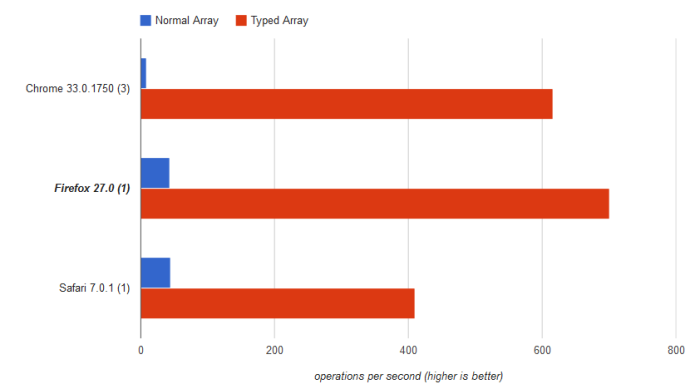# Efficiency of 2D Typed Arrays vs Normal Arrays

Posted on February, 22 2014

The efficiency of some aspects of JavaScript surprise me, in good ways and bad ways.

When working on my latest project, It was originally using the normal Array that JavaScript provides, since it was the most straight-forward option. The project required to use a 2D array to store points on a grid.

The method used to create a 2D array was by nesting Arrays with two coordinates in one big array:

``````var array = [[x0,y0],[x1,x2]];
array[i] = x;
array[i] = y;``````

Originally I thought this method was fine and dandy until I realized that the algorithm is extremely slow... Looking over it I saw nothing that I could improve so I just set it back into the corner for a bit.

Flash-forward a week, and I find the existence of Typed Arrays.

What's so special about Typed Arrays? Well, there's a myriad of differences between Typed arrays and normal arrays. First off, with normal JavaScript Arrays, they're not actually arrays. What I mean by this is that JavaScript arrays are actually just key-value objects, and the indexes are not integers, they're strings- generally. JavaScript engines do different things with Arrays, and that's where JavaScript gets its unreliable performance from. Typed Arrays on the other hand uses a set chunk of memory to construct a C-style array with a variety of different data Types (and yes, this does mean that all numbers in JavaScript that aren't doubles).

Using 2D Typed Arrays is a bit more complex initially. First, nesting Typed Arrays is pointless, since you're still using the wonky normal arrays. Therefore, we must create a flat array that's x*y big. For a Boolean Typed Array we will use an Unsigned 8 bit Array:

``var array = new Uint8Array(Array(x*y))``

To read/write to this array, we must assume how the data is stored. In languages like C, this is generally implemented in a colexicographic order (also known as Row-major order), likewise in other languages like Fortran use a lexicographic ordering (also known as Column-major order). Assuming a colexicographic order, we can calculate an offset based on X Y coordinates, like so:

``index = (columns * x) + y``

With this offset calculated, all we have to do is throw it as an index in our typed array in one neat little statement:

``````//maxy is the maximum Y value
array[maxy*x+y] = 1;``````

Originally I was a tad naive on the speed, so I ran some tests. The results I got were shocking, since I knew the normal arrays in JavaScript were bad but... that bad?This test did 1 basic operation: go through a 1,000^2 2D array and set all the values to one.

## Setup:

``````var normal = [];
var typed = new Uint8Array(new Array(1000*1000));``````

## Normal Array:

``````for (var x = 0; x < 1000; x++) {
for (var y = 0; y < 1000; y++) {
normal.push([x,y]);
}
}``````

## Typed Array:

``````for (var x = 0; x < 1000; x++) {
for (var y = 0; y < 1000; y++) {
typed[1000*x+y] = 1;
}
}``````

The normal array was 95% slower than the typed array on average. To put that in perspective, the normal array did 32 operations per second on average. The typed array on the other hand did 575 operations/second on average, leaving it 18x faster than the normal array.

In hindsight, I feel pretty dumb to even use my original method.